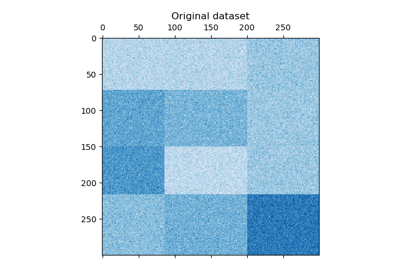# sklearn.cluster.SpectralBiclustering¶

class sklearn.cluster.SpectralBiclustering(n_clusters=3, method='bistochastic', n_components=6, n_best=3, svd_method='randomized', n_svd_vecs=None, mini_batch=False, init='k-means++', n_init=10, n_jobs=None, random_state=None)[source]

Spectral biclustering (Kluger, 2003).

Partitions rows and columns under the assumption that the data has an underlying checkerboard structure. For instance, if there are two row partitions and three column partitions, each row will belong to three biclusters, and each column will belong to two biclusters. The outer product of the corresponding row and column label vectors gives this checkerboard structure.

Read more in the User Guide.

Parameters
n_clustersint or tuple (n_row_clusters, n_column_clusters), default=3

The number of row and column clusters in the checkerboard structure.

method{‘bistochastic’, ‘scale’, ‘log’}, default=’bistochastic’

Method of normalizing and converting singular vectors into biclusters. May be one of ‘scale’, ‘bistochastic’, or ‘log’. The authors recommend using ‘log’. If the data is sparse, however, log normalization will not work, which is why the default is ‘bistochastic’.

Warning

if method='log', the data must be sparse.

n_componentsint, default=6

Number of singular vectors to check.

n_bestint, default=3

Number of best singular vectors to which to project the data for clustering.

svd_method{‘randomized’, ‘arpack’}, default=’randomized’

Selects the algorithm for finding singular vectors. May be ‘randomized’ or ‘arpack’. If ‘randomized’, uses randomized_svd, which may be faster for large matrices. If ‘arpack’, uses scipy.sparse.linalg.svds, which is more accurate, but possibly slower in some cases.

n_svd_vecsint, default=None

Number of vectors to use in calculating the SVD. Corresponds to ncv when svd_method=arpack and n_oversamples when svd_method is ‘randomized.

mini_batchbool, default=False

Whether to use mini-batch k-means, which is faster but may get different results.

init{‘k-means++’, ‘random’} or ndarray of (n_clusters, n_features), default=’k-means++’

Method for initialization of k-means algorithm; defaults to ‘k-means++’.

n_initint, default=10

Number of random initializations that are tried with the k-means algorithm.

If mini-batch k-means is used, the best initialization is chosen and the algorithm runs once. Otherwise, the algorithm is run for each initialization and the best solution chosen.

n_jobsint, default=None

The number of jobs to use for the computation. This works by breaking down the pairwise matrix into n_jobs even slices and computing them in parallel.

None means 1 unless in a joblib.parallel_backend context. -1 means using all processors. See Glossary for more details.

random_stateint, RandomState instance, default=None

Used for randomizing the singular value decomposition and the k-means initialization. Use an int to make the randomness deterministic. See Glossary.

Attributes
rows_array-like of shape (n_row_clusters, n_rows)

Results of the clustering. rows[i, r] is True if cluster i contains row r. Available only after calling fit.

columns_array-like of shape (n_column_clusters, n_columns)

Results of the clustering, like rows.

row_labels_array-like of shape (n_rows,)

Row partition labels.

column_labels_array-like of shape (n_cols,)

Column partition labels.

References

Examples

>>> from sklearn.cluster import SpectralBiclustering
>>> import numpy as np
>>> X = np.array([[1, 1], [2, 1], [1, 0],
...               [4, 7], [3, 5], [3, 6]])
>>> clustering = SpectralBiclustering(n_clusters=2, random_state=0).fit(X)
>>> clustering.row_labels_
array([1, 1, 1, 0, 0, 0], dtype=int32)
>>> clustering.column_labels_
array([0, 1], dtype=int32)
>>> clustering
SpectralBiclustering(n_clusters=2, random_state=0)


Methods

 fit(self, X[, y]) Creates a biclustering for X. get_indices(self, i) Row and column indices of the i’th bicluster. get_params(self[, deep]) Get parameters for this estimator. get_shape(self, i) Shape of the i’th bicluster. get_submatrix(self, i, data) Return the submatrix corresponding to bicluster i. set_params(self, \*\*params) Set the parameters of this estimator.
__init__(self, n_clusters=3, method='bistochastic', n_components=6, n_best=3, svd_method='randomized', n_svd_vecs=None, mini_batch=False, init='k-means++', n_init=10, n_jobs=None, random_state=None)[source]

Initialize self. See help(type(self)) for accurate signature.

property biclusters_

Convenient way to get row and column indicators together.

Returns the rows_ and columns_ members.

fit(self, X, y=None)[source]

Creates a biclustering for X.

Parameters
Xarray-like, shape (n_samples, n_features)
yIgnored
get_indices(self, i)[source]

Row and column indices of the i’th bicluster.

Only works if rows_ and columns_ attributes exist.

Parameters
iint

The index of the cluster.

Returns
row_indnp.array, dtype=np.intp

Indices of rows in the dataset that belong to the bicluster.

col_indnp.array, dtype=np.intp

Indices of columns in the dataset that belong to the bicluster.

get_params(self, deep=True)[source]

Get parameters for this estimator.

Parameters
deepbool, default=True

If True, will return the parameters for this estimator and contained subobjects that are estimators.

Returns
paramsmapping of string to any

Parameter names mapped to their values.

get_shape(self, i)[source]

Shape of the i’th bicluster.

Parameters
iint

The index of the cluster.

Returns
shape(int, int)

Number of rows and columns (resp.) in the bicluster.

get_submatrix(self, i, data)[source]

Return the submatrix corresponding to bicluster i.

Parameters
iint

The index of the cluster.

dataarray

The data.

Returns
submatrixarray

The submatrix corresponding to bicluster i.

Notes

Works with sparse matrices. Only works if rows_ and columns_ attributes exist.

set_params(self, **params)[source]

Set the parameters of this estimator.

The method works on simple estimators as well as on nested objects (such as pipelines). The latter have parameters of the form <component>__<parameter> so that it’s possible to update each component of a nested object.

Parameters
**paramsdict

Estimator parameters.

Returns
selfobject

Estimator instance.

## Examples using sklearn.cluster.SpectralBiclustering`¶